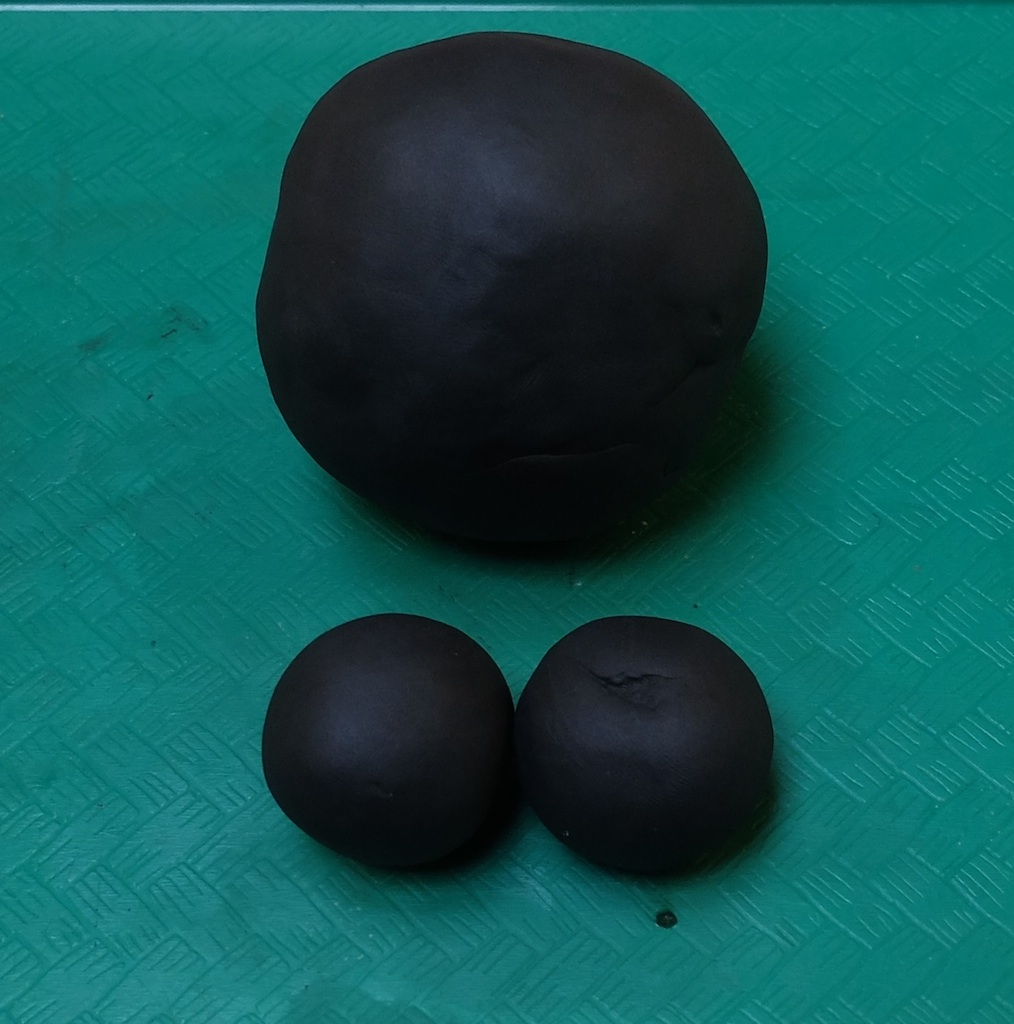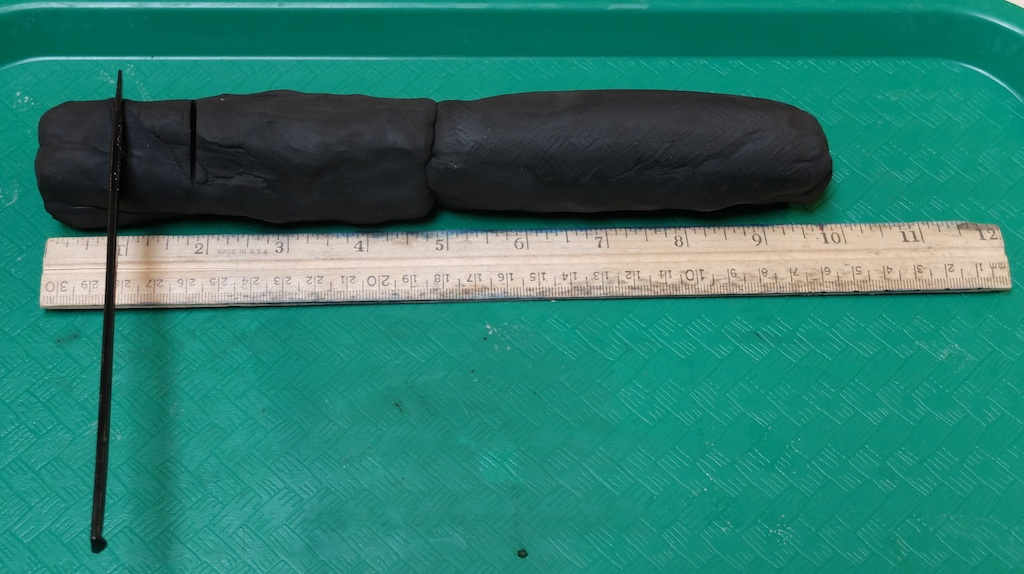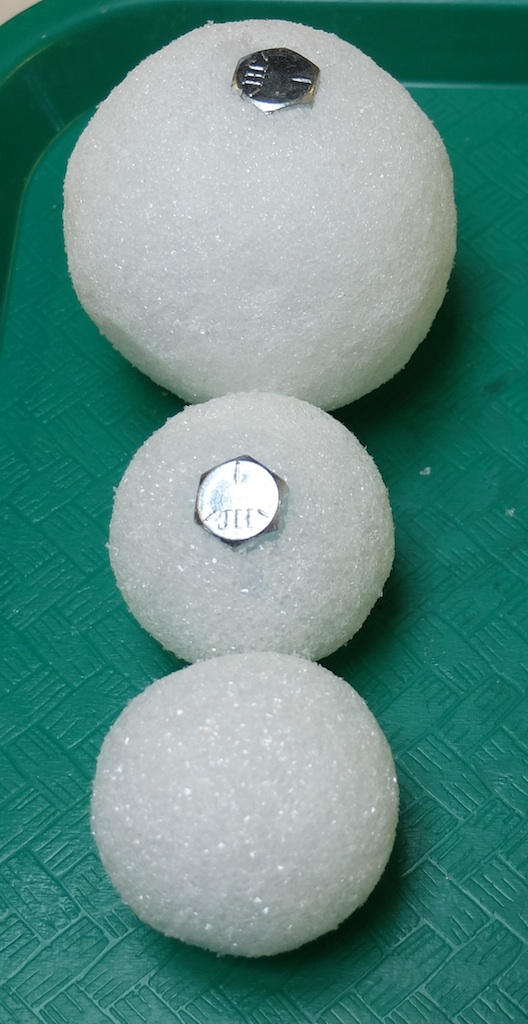When black holes collide.Two black holes before they collide and one after

Introduction

Use clay to model what happens when two black holes collide.

Material

• Clay or Playdoh
• A plastic knife
• A ruler.

Optional

• Two Styrofoam balls with radius 1 inch (2.5 cm) (any reasonable size will do)
• One Styrofoam ball with twice the radius of the two balls above.
• Four bolts, about 0.75 inch long and 1/4 inch diameter. (They should fit into a hole you drill in the Styrofoam sphere)

To Do and Notice

Roll the clay into a cylinder.Cut the cylinder into three pieces one piece 0.8 of the cylinder length, and two pieces 0.1 of the cylinder length.

Roll the three segments into spheres. (See image at the top of the page.)

If the radius of the small spheres is r = 1, then the radius of the big sphere is R = 2.

What's Going On?

The radius of a black hole is proportional to its mass, M.

r = 2 GM/c^2

G is the universal gravitational constant

c is the speed of light.

When two black holes with the same mass and therefor radius collide and combine the mass of the resulting black hole is double that of each of the original black holes, so the radius of the new black hole is double that of the original black holes.

This shows how weird black holes are, if you collide two spheres of clay the resulting sphere has twice the mass and a radius of only the cube root of 2 about 1.26 times the radius. In addition your sphere with twice the radius has eight times the mass of each sphere not just twice.

Going Further

To make a slightly better model drill a hole into each of the smaller Styrofoam balls and push a bolt into the hole.(Use water based or hot melt glue if needed.)Drill two holes on opposite sides of the larger sphere and push one bolt into each hole.

When you are done you will have two balls of mass 1 and a ball with twice the radius of mass 2. (Since the Styrofoam weighs so little compared to the weight of the bolts.)

Math Root

The volume, V, of a sphere of radius R is

V = 4/3piR3

Etc.

What would be the mass of a black hole with a radius of 2 inches, or 5 cm?

M = r c^2/2 G = 0.05 x (3 x 10^8)^2 / 6.67 x 10^-11 = 10^26 kg about 10 times the mass of the earth.

 Scientific Explorations by Paul Doherty © 2014 3 May 2014